Schwarzschild Black Hole

Schwarzschild coordinates

The spacetime metric is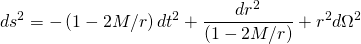where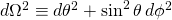is the metric for the unit two-sphere. The horizon is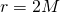and the singularity is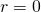. The lapse function, shift vector, and extrinsic curvature defined by the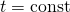slices and time flow vector field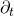are:

(1)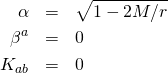The geodesic equations are:

Isotropic coordinates

Starting with Schwarzschild coordinates, the transformationgives the line element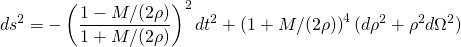The horizon is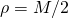. The lapse function, shift vector, and extrinsic curvature defined by theslices and the time flow vector fieldare:

(2)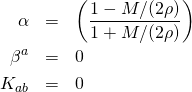The geodesic equations are:

Kerr-Schild coordinates

Starting with Schwarzschild coordinates, the transformation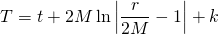gives the line element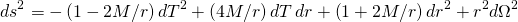The lapse function, shift vector, and extrinsic curvature defined by the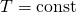slices and the time flow vector fieldare:

(3)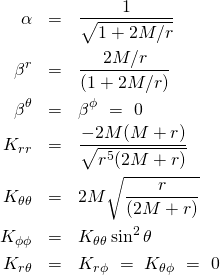Changing from spherical coordinates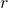,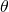,to Cartesian coordinates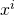givesThis can also be written aswhere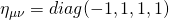is the Minkowski metric,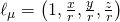is a covector, and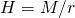. Also let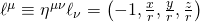. Then the inverse metric is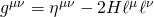. Note that the vector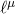is null in both the Minkowski and physical metrics.

The geodesic equations:

Kruskal coordinates

Starting with Schwarzschild coordinates, let

(4)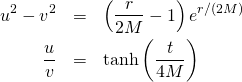The line element becomes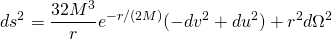The lapse function, shift vector, and extrinsic curvature defined by the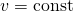slices and the time flow vector field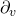are:

(5)Geodesic equations: